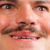# Hard Would You Rather

## This is a would you rather game... but with hard questions!On Apr 18, 2019### Would You Rather

Calculating results### Would You Rather...

Calculating results### Would You Rather...

Calculating results### Would You Rather...

Calculating results### Would You Rather...

Calculating results### Would You Rather...

Calculating results### Would You Rather...

Calculating results### Would You Rather...

Calculating results### Would You Rather...

Calculating results### Would You Rather...

Calculating results### Would You Rather...

Calculating results### Would You Rather...

Calculating results### Would You Rather...

Calculating results### Would You Rather...

Calculating results### Would You Rather...

Calculating results### Would You Rather...

Calculating results### Would You Rather...

Calculating results### Would You Rather...

Calculating results### Would You Rather...

Calculating results### Would You Rather...

Calculating results### Would You Rather...

Calculating results### Would You Rather...

Calculating results

### If you like stupid, funny stuff, subscribe to my channel!https://m.youtube.com/channel/UCj4nNBUjjnXbrpgEbXiQH4g### Did You Like the Would You Rather?

Calculating results